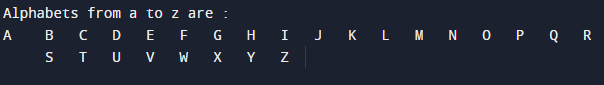# C Program to print Alphabets from A-Z

In this tutorial you will learn about the C Program to print Alphabets from A-Z and its application with practical example.

In this tutorial, we will learn to create a C program which will print alphabet  form A-Z(Capital) using C programming.

## Prerequisites

Before starting with this tutorial we assume that you are best aware of the following C programming topics:

• Data type
• C  loops.

## Program to Print Alphabets from A-Z.

This a program to print the English alphabets (A-Z) is very simple. The following code is used to print English alphabets form (A-Z).Here we are going to create a simple c program in which we will display how a alphabet program code and logic works lets begun with our code.

## C Program to Print Alphabets from A-Z.

In this program we will print complete series from A-Z using for loop.
Firstly we declare required header file and variable.It is not as  complicated as you imagined. Let’s see the code.

## OutputIn the above program, we have first declared and initialized a set variables required in the program.

• alpha= for holding value from A-Z.

Here we creating a program in which we print series from ‘A-Z’

Here first we take a variable name alpha and Initialize loop  variable from alpha=’A’, that goes till alpha=’Z ‘, then increment the loop each iteration by 1. The structure of loop look like thiswithin the loop we print the value of alpha and we will get the following series.In this program, the loop display the English alphabet in Uppercase.

In this tutorial we have learn about the C Program to print Alphabets from A-Z and its application with practical example. I hope you will like this tutorial.